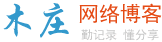# 用C#实现阶乘运算

```public static double JieCheng(int number)
{
if (number == 0)
{
return 0;
}

//初始值必须设置为1
double result = 1;

for (int i = number; i >= 1; i--)
{
result = result*i;
}
return result;
}``````public static double JieCheng(int number)
{
if (number == 0)
{
return 1;
}

return number * JieCheng(number - 1);
}```### 评论

• 欢迎访问木庄网络博客
• 可复制：代码框内的文字。
• 方法：Ctrl+C。